# Munkres’ Assignment Algorithm

Modified for Rectangular Matrices

Assignment Problem – Let C be an nxn matrix representing the costs of each of n workers to perform any of n jobs.  The assignment problem is to assign jobs to workers so as to minimize the total cost. Since each worker can perform only one job and each job can be assigned to only one worker the assignments constitute an independent set of the matrix C.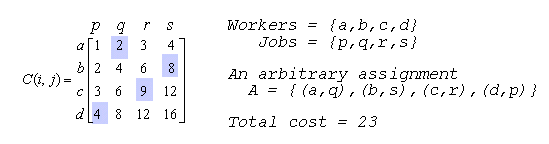An arbitrary assignment is shown above in which worker a is assigned job q, worker b is assigned job s and so on.  The total cost of this assignment is 23.  Can you find a lower cost assignment? Can you find the minimal cost assignment? Remember that each assignment must be unique in its row and column.

A brute-force algorithm for solving the assignment problem involves generating all independent sets of the matrix C, computing the total costs of each assignment and a search of all assignment to find a minimal-sum independent set. The complexity of this method is driven by the number of independent assignments possible in an nxn matrix. There are choices for the first assignment, n-1 choices for the second assignment and so on, giving n! possible assignment sets. Therefore, this approach has, at least, an exponential runtime complexity.As each assignment is chosen that row and column are eliminated from consideration.  The question is raised as to whether there is a better algorithm.  In fact there exists a polynomial runtime complexity algorithm for solving the assignment problem developed by James Munkre’s in the late 1950’s despite the fact that some references still describe this as a problem of exponential complexity.

The following 6-step algorithm is a modified form of the original Munkres’ Assignment Algorithm (sometimes referred to as the Hungarian Algorithm).  This algorithm describes to the manual manipulation of a two-dimensional matrix by starring and priming zeros and by covering and uncovering rows and columns.  This is because, at the time of publication (1957), few people had access to a computer and the algorithm was exercised by hand.
Step 0:  Create an nxm  matrix called the cost matrix in which each element represents the cost of assigning one of n workers to one of m jobs.  Rotate the matrix so that there are at least as many columns as rows and let k=min(n,m).

Step 1:  For each row of the matrix, find the smallest element and subtract it from every element in its row.  Go to Step 2.

Step 2:  Find a zero (Z) in the resulting matrix.  If there is no starred zero in its row or column, star Z. Repeat for each element in the matrix. Go to Step 3.

Step 3:  Cover each column containing a starred zero.  If K columns are covered, the starred zeros describe a complete set of unique assignments.  In this case, Go to DONE, otherwise, Go to Step 4.

Step 4:  Find a noncovered zero and prime it.  If there is no starred zero in the row containing this primed zero, Go to Step 5.  Otherwise, cover this row and uncover the column containing the starred zero. Continue in this manner until there are no uncovered zeros left. Save the smallest uncovered value and Go to Step 6.

Step 5:  Construct a series of alternating primed and starred zeros as follows.  Let Z0 represent the uncovered primed zero found in Step 4.  Let Z1 denote the starred zero in the column of Z0 (if any). Let Z2 denote the primed zero in the row of Z1 (there will always be one).  Continue until the series terminates at a primed zero that has no starred zero in its column.  Unstar each starred zero of the series, star each primed zero of the series, erase all primes and uncover every line in the matrix.  Return to Step 3.

Step 6:  Add the value found in Step 4 to every element of each covered row, and subtract it from every element of each uncovered column.  Return to Step 4 without altering any stars, primes, or covered lines.

DONE:  Assignment pairs are indicated by the positions of the starred zeros in the cost matrix.  If C(i,j) is a starred zero, then the element associated with row i is assigned to the element associated with column j.

Some of these descriptions require careful interpretation.  In Step 4, for example, the possible situations are, that there is a noncovered zero which get primed and if there is no starred zero in its row the program goes onto Step 5.  The other possible way out of Step 4 is that there are no noncovered zeros at all, in which case the program goes to Step 6.

At first it may seem that the erratic nature of this algorithm would make its implementation difficult.  However, we can apply a few general rules of programming style to simplify this problem.  The same rules can be applied to any step-algorithm.

Good Programming Style and Design Practices

1. Strive to create readable source code through the use of blank lines, comments and spacing.

2. Use consistent naming conventions, for variable and constant identifiers and subprograms.

3. Use consistent indentation and always indent the bodies of conditionals and looping constructs.

4. Place logically distinct computations in their own execution blocks or in separate subprograms.

5. Don’t use global variables inside subprograms except where such use is clear and improves readability and efficiency.

6. Use local variables where appropriate and try to limit the creation of unnecessary identifiers in the main program.

7. Open I/O files only when needed and close them as soon as they are no longer required.

8. Work to keep the level of nesting of conditionals and loops at a minimum.

9. Use constant identifiers instead of hardwiring for-loop and array ranges in the body of the code with literal values.

10. When you feel that things are getting out of control, start over. Re-coding is good coding.

An Implementation of Munkres’ Assignment Algorithm

By applying Rule 4 to the step-algorithm we decide to make each step its own procedure.  Now we can apply Rule 8 by using a case statement in a loop to control the ordering of step execution.  The main loop for Munkres as a step-wise algorithm is shown here implemented in C#.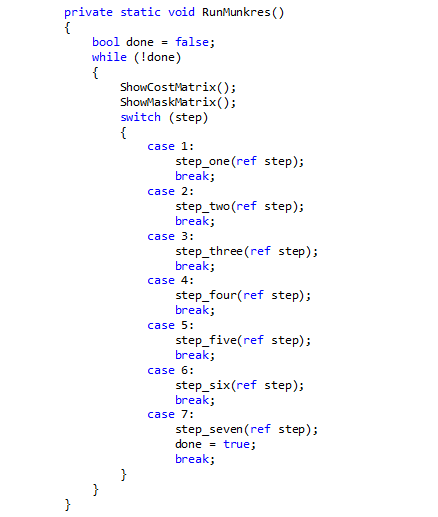In each pass of the loop the step procedure called sets the value of step for the next pass.  When the algorithm is finished the value of step is set to some value outside the range 1..7 so that done will be set to true and the program will end.  In the completed program the tagged (starred) zeros flag the row/column pairs that have been assigned to each other.  We will discuss the implementation of a procedure for each step of Munkres’ Algorithm below. We will assume that the cost matrix C(i,j) has already been loaded with the first index referring to the row number and the second index referring to the column number.

Step 1
For each row of the matrix, find the smallest element and subtract it from every element in its row.  Go to Step 2. We can define a local variable called minval that is used to hold the smallest value in a row.  Notice that there are two loops over the index j appearing inside an outer loop over the index i. The first inner loop over the index j searches a row for the minval.  Once minval has been found this value is subtracted from each element of that row in the second inner loop over j.  The value of step is set to 2 just before stepone ends.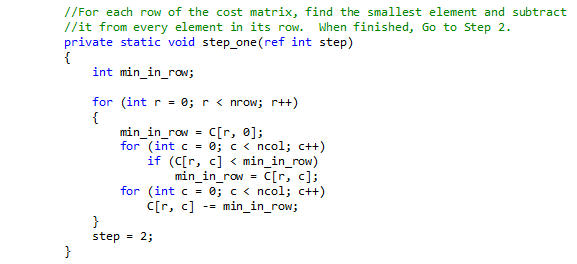Step 2
Find a zero (Z) in the resulting matrix.  If there is no starred zero in its row or column, star Z. Repeat for each element in the matrix. Go to Step 3.  In this step, we introduce the mask matrix M, which in the same dimensions as the cost matrix and is used to star and prime zeros of the cost matrix.  If M(i,j)=1 then C(i,j) is a starred zero,  If M(i,j)=2 then C(i,j) is a primed zero.  We also define two vectors R_cov and C_cov that are used to “cover” the rows and columns of the cost matrix C.  In the nested loop (over indices i and j) we check to see if C(i,j) is a zero value and if its column or row is not already covered.  If not then we star this zero (i.e. set M(i,j)=1) and cover its row and column (i.e. set R_cov(i)=1 and C_cov(j)=1).  Before we go on to Step 3, we uncover all rows and columns so that we can use the cover vectors to help us count the number of starred zeros.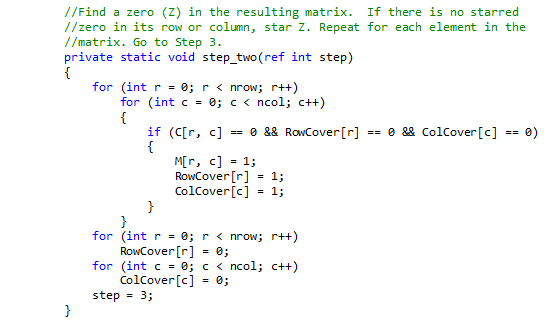Step 3
Cover each column containing a starred zero.  If K columns are covered, the starred zeros describe a complete set of unique assignments.  In this case, Go to DONE, otherwise, Go to Step 4. Once we have searched the entire cost matrix, we count the number of independent zeros found.  If we have found (and starred) K independent zeros then we are done.  If not we procede to Step 4.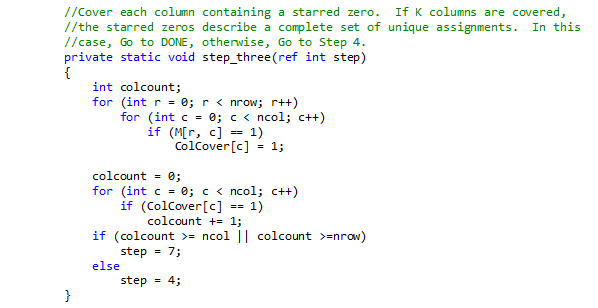Step 4

Find a noncovered zero and prime it.  If there is no starred zero in the row containing this primed zero, Go to Step 5.  Otherwise, cover this row and uncover the column containing the starred zero. Continue in this manner until there are no uncovered zeros left. Save the smallest uncovered value and Go to Step 6.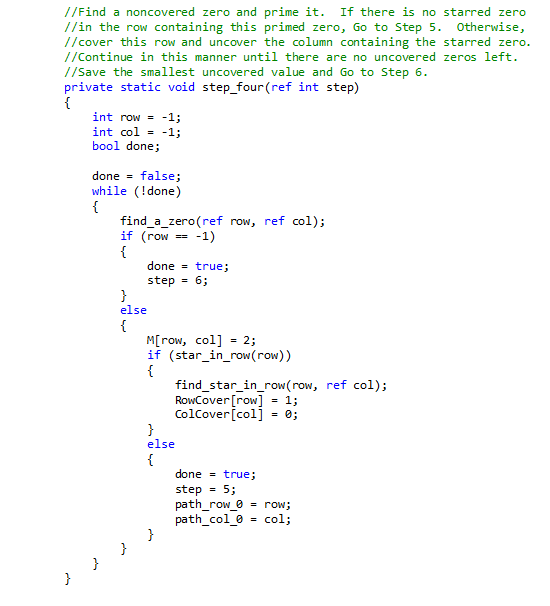In this step, statements such as “find a noncovered zero” are clearly distinct operations that deserve their own functional blocks.  We have decomposed this step into a main method and three subprograms (2 methods and a boolean function).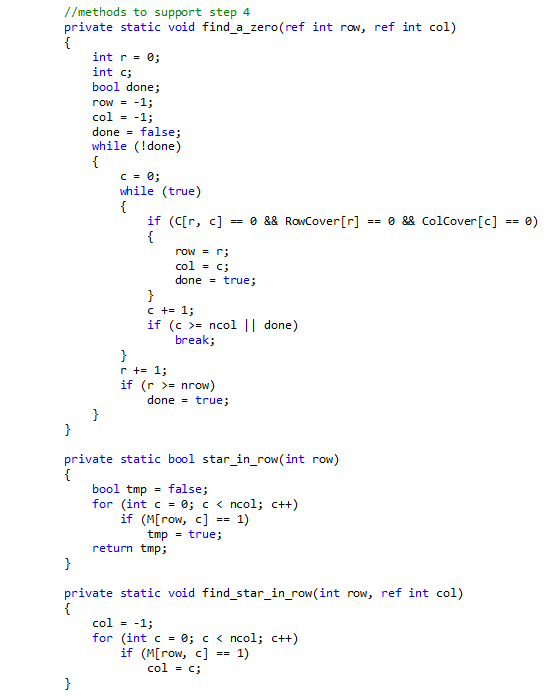Step 5
Construct a series of alternating primed and starred zeros as follows.  Let Z0 represent the uncovered primed zero found in Step 4.  Let Z1 denote the starred zero in the column of Z0 (if any). Let Z2 denote the primed zero in the row of Z1 (there will always be one).  Continue until the series terminates at a primed zero that has no starred zero in its column.  Unstar each starred zero of the series, star each primed zero of the series, erase all primes and uncover every line in the matrix.  Return to Step 3.  You may notice that Step 5 seems vaguely familiar.  It is a verbal description of the augmenting path algorithm (for solving the maximal matching problem) which we discussed in Lecture 3.  We decompose the operations of this step into a main procedure and five relatively simple subprograms.As with the previous step we have decomposed Step 5 into a number of separate methods each performing a functionally distinct task corresponding to the description in Munkres algorithm.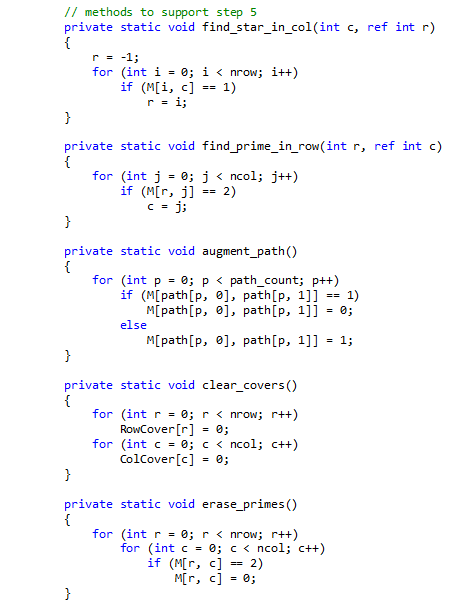Step 6
Add the value found in Step 4 to every element of each covered row, and subtract it from every element of each uncovered column.  Return to Step 4 without altering any stars, primes, or covered lines. Notice that this step uses the smallest uncovered value in the cost matrix to modify the matrix.  Even though this step refers to the value being found in Step 4 it is more convenient to wait until you reach Step 6 before searching for this value.  It may seem that since the values in the cost matrix are being altered, we would lose sight of the original problem.  However, we are only changing certain values that have already been tested and found not to be elements of the minimal assignment.  Also we are only changing the values by an amount equal to the smallest value in the cost matrix, so we will not jump over the optimal (i.e. minimal assignment) with this change.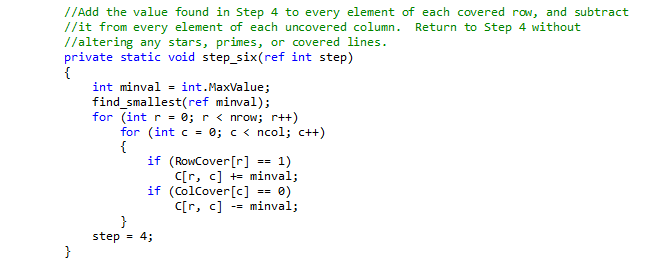For Step 6, there is one supporting method to find the smallest uncovered value (described in Step 4).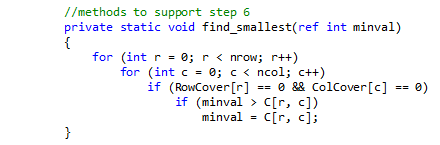The code used above is available in a complete Microsoft Visual Studio .NET 2010 C# project – Munkres.  This is a console application that can read a text-file containing the cost matrix values arranged in a two-dimensional array or generate test matrices.  The input file should be placed in the same folder as the calling executable.  This project application can generate a random value cost matrix or a worst-case test matrix C(i,j) = i*j.  Setting the value of each element C(i,j) of the cost matrix to the value i*j ensures that the maximum number of operations will be required to find the minimal assignment (which is the back diagonal).  The locations of the ones (1’s) in the associated mask matrix M correspond to the assignment pairs selected by Munkres’ Algorithm.  The following is an example run of the algorithm on a 3×3 worst-case test matrix.

## An Example Execution of Munkres’ Algorithm

Workers = { a, b, c}
Jobs = {p, q, r}

Cost of assigning job j to work i is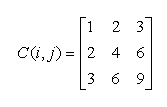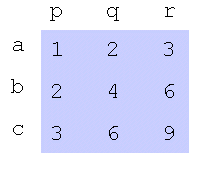1. Step 0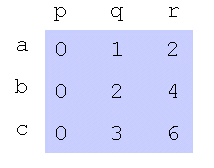2. Step 1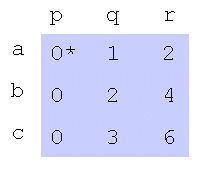3. Step 2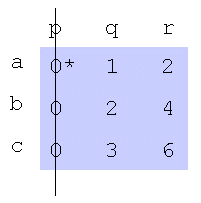4. Step 3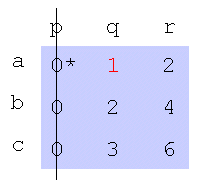5. Step 4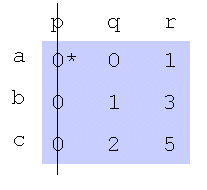6. Step 6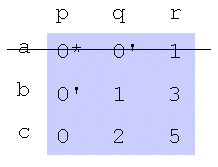7. Step 4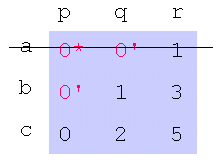8. Step 5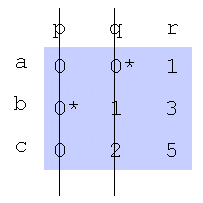9. Step 3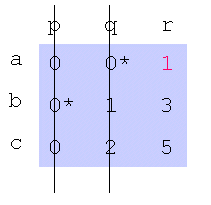10. Step 4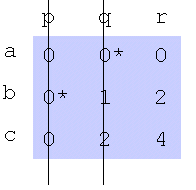11. Step 6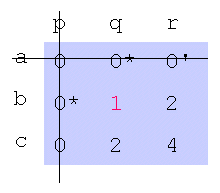12. Step 4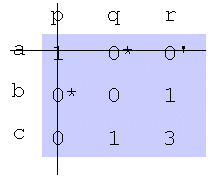13. Step 6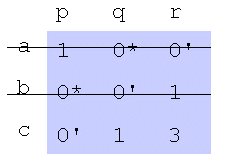14. Step 4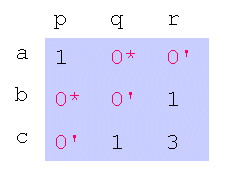15. Step 5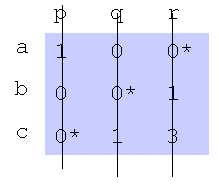16. Step 317. Done

This example illustrates the method of implementing a step-algorithm.  It also serves to demonstrate why we do not attempt to implement every algorithm discussed in this course. 🙂

1. The algorthm will work even when the minimum values in two or more rows are in the same column.

2. The algorithm will work even when two or more of the rows contain the same values in the the same order.

3. The algorithm will work even when all the values are the same (although the result is not very interesting).

4. Munkres Assignment Algorithm is not exponential run time or intractable; Munkres can achieve a low order polynomial run time, worst-case O(n3). see doi: 10.1007/BF01930994

5. Optimality is guaranteed in Munkres Assignment Algorithm.

6. Setting the cost matrix to C(i,j) = i*j  makes a good testing matrix for this problem.

7. In this algorithm the range of indices is[0..n-1] rather than [1..n].

8. Step 3 is an example of the greedy method.  If the minimum values are all in different rows then their positions represent the minimal pairwise assignments.

9. Step 5 is an example of the Augmenting Path Algorithm (Stable Marriage Problem).

10. Step 6 is an example of constraint relaxation.  It is “giving up” on a particular cost and raising the constraint by the least amount possible.

11. If your implementation is jumping between Step 4 and Step 6 without entering Step 5, you probably have not properly dealt with recognizing that there are no uncovered zeros in Step 4.

12. In the matrix M 1=starred zero and 2=primed zero.  So, if C[i,j] is a starred zero we would set M[i,j]=1.  All other elements in M are set to zero

13. The Munkres assignment algorithm can be implemented as a sparse matrix, but you will need to ensure that the correct (optimal) assignment pairs are active in the sparse cost matrix C

14. Munkres Assignment can be applied to TSP, pattern matching, track initiation, data correlation, and (of course) any pairwise assignment application.

15. Munkres can be extended to rectangular arrays (i.e. more jobs than workers, or more workers than jobs).

16. The best way to find a maximal assignment is to replace the values ci,j in the cost matrix with C[i,j] = bigval – ci,j.

17. Original Reference:  Algorithms for Assignment and Transportation Problems, James Munkres, Journal of the Society for Industrial and Applied Mathematics Volume 5, Number 1, March, 1957

18. Extension to Rectangular Arrays Ref:  F. Burgeois and J.-C. Lasalle. An extension of the Munkres algorithm for the assignment problem to rectangular matrices. Communications of the ACM, 142302-806, 1971.